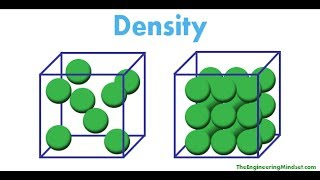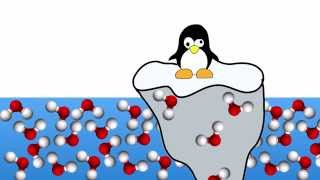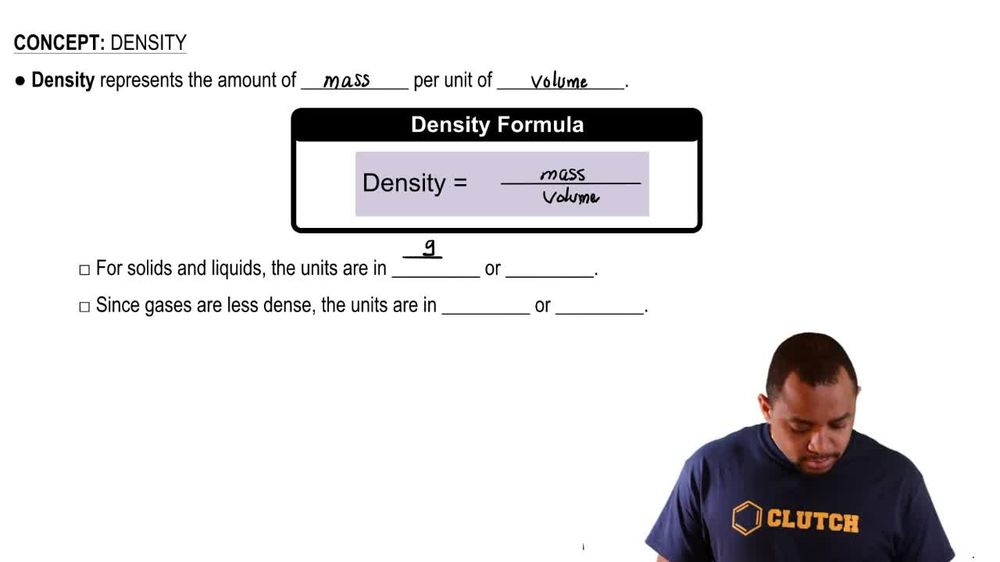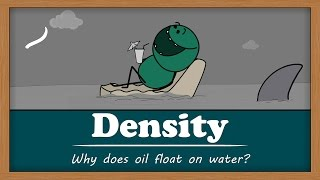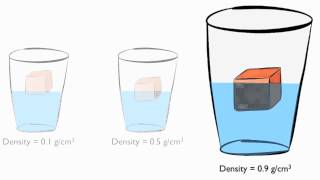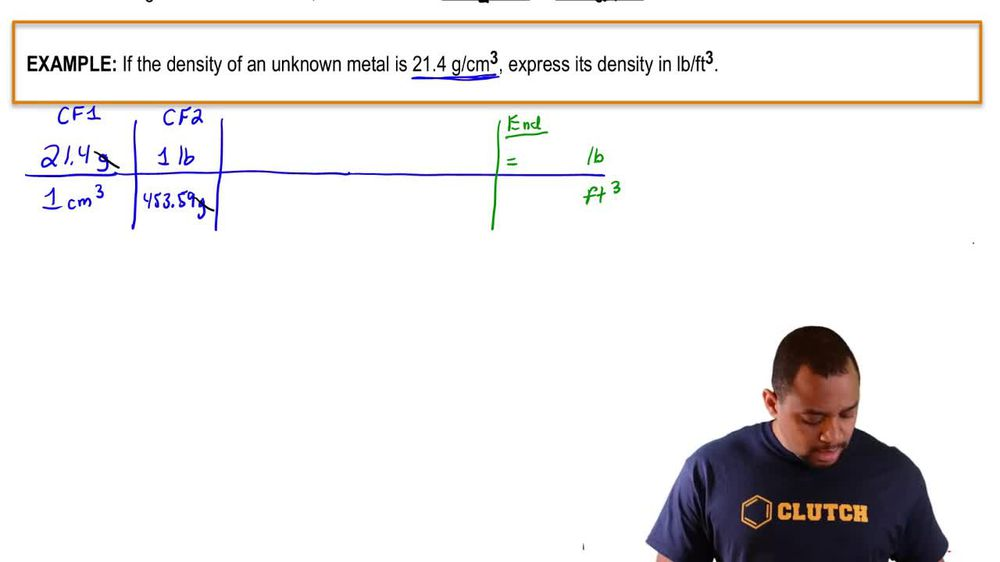Start typing, then use the up and down arrows to select an option from the list.
1. 1. Intro to General Chemistry2. Density
Problem

# (b) Three cubes of equal mass are composed of gold 1density = 19.32 g/cm32, platinum 1density = 21.45 g/cm32, and lead 1density = 11.35 g/cm32. List the cubes from smallest to largest.

Relevant Solution3m
Play a video:
Hi everyone here we have a problem telling asking us to list a potassium cube density equals 0.862 g per centimeters cube. A cesium cube density equals 1.9 g per centimeters cube. And rubidium cube density equals 1.63 g per centimeter cube that have similar masses from smallest to largest. So density equals mass over volume. We know all of their densities and we know that their masses are similar. So we want to solve for volume. So we're going to multiply both sides by volume to cancel that out, which gives us density times volume equals mass. So then we want to divide both sides by density to cancel that out. And I sleep volume. So we get volume equals mass over density. So we're going to assume that each cube is one g. So for potassium We have one g Divided by 0.862 g per cm cube. So our grams are going to cancel out here And that's going to give us 1.16 cm cute. We have cesium Which equals one g Divided by 1.9 grams per centimeters cubed. And our g cancel out. And that gives us 0.52 cm cubed. And lastly we have rubidium Which is one g divided by 1.63 g per centimeters cubed. And our grams cancel out that equals 0. centimeters cubed. So now we just have to list the smallest to largest. So we have cesium is less than rubidium is less than potassium. And that is our final answer. Thank you for watching Bye!